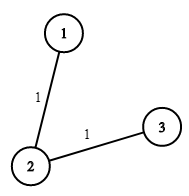1168. Optimize Water Distribution in a Village

There are `n` houses in a village. We want to supply water for all the houses by building wells and laying pipes.

For each house `i`, we can either build a well inside it directly with cost `wells[i - 1]` (note the `-1` due to 0-indexing), or pipe in water from another well to it. The costs to lay pipes between houses are given by the array `pipes`, where each `pipes[j] = [house1j, house2j, costj]` represents the cost to connect `house1j` and `house2j` together using a pipe. Connections are bidirectional.

Return the minimum total cost to supply water to all houses.

Example 1:```Input: n = 3, wells = [1,2,2], pipes = [[1,2,1],[2,3,1]]
Output: 3
Explanation:
The image shows the costs of connecting houses using pipes.
The best strategy is to build a well in the first house with cost 1 and connect the other houses to it with cost 2 so the total cost is 3.
```

Constraints:

• `1 <= n <= 104`
• `wells.length == n`
• `0 <= wells[i] <= 105`
• `1 <= pipes.length <= 104`
• `pipes[j].length == 3`
• `1 <= house1j, house2j <= n`
• `0 <= costj <= 105`
• `house1j != house2j`

1168. Optimize Water Distribution in a Village
``````struct Solution;
use std::cmp::Reverse;
use std::collections::BinaryHeap;

struct UnionFind {
parent: Vec<usize>,
n: usize,
}

impl UnionFind {
fn new(n: usize) -> Self {
let parent = (0..n).collect();
UnionFind { parent, n }
}

fn find(&mut self, i: usize) -> usize {
let j = self.parent[i];
if i != j {
let k = self.find(j);
self.parent[i] = k;
k
} else {
j
}
}

fn union(&mut self, i: usize, j: usize) -> bool {
let i = self.find(i);
let j = self.find(j);
if i != j {
self.parent[i] = j;
true
} else {
false
}
}
}

impl Solution {
fn min_cost_to_supply_water(n: i32, wells: Vec<i32>, pipes: Vec<Vec<i32>>) -> i32 {
let n = n as usize + 1;
let mut queue: BinaryHeap<(Reverse<i32>, usize, usize)> = BinaryHeap::new();
for i in 0..n - 1 {
queue.push((Reverse(wells[i]), 0, i + 1));
}
for pipe in pipes {
queue.push((Reverse(pipe), pipe as usize, pipe as usize));
}
let mut res = 0;
let mut uf = UnionFind::new(n);
while let Some(e) = queue.pop() {
let u = e.1;
let v = e.2;
if uf.union(u, v) {
res += (e.0).0;
}
}
res
}
}

#[test]
fn test() {
let n = 3;
let wells = vec![1, 2, 2];
let pipes = vec_vec_i32![[1, 2, 1], [2, 3, 1]];
let res = 3;
assert_eq!(Solution::min_cost_to_supply_water(n, wells, pipes), res);
}
``````